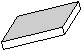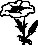## NCERT 6TH CLASS MATHEMATICS CHAPTER DATA HANDLING EXERCISE

### OBJECTIVE :

1. C 2. C 3. A 4. C 5. D 6. C 7. D

8. A 9. A 10. A 11. C 12. D

SUBJECTIVE :
1. (i) maximum number of families with different number of members in family
(ii) 50
(iii) 3, 120
(iv) 8, 9, 10; 10

5. Monday (10), Tuesday (15), Wednesday(10), Thursday (20), Friday(15)

6. (a) 48 (b) Water park
(c) Sea side (d) 16

8. (a) 18000 (b) 14000
(c) 2007

In questions 1 to 5, out of the four options, only one is correct. write the correct answer.
1. Using tally marks, which one of the following represents the number eight:2. The marks (out of 10) obtained by 28 students in a Mathematics test are listed as below:
8, 1, 2, 6, 5, 5, 5, 0, 1, 9, 7, 8, 0, 5, 8, 3, 0, 8, 10, 10, 3, 4, 8, 7, 8, 9,2, 0
The number of students who obtained marks more than or equal to 5 is
(A) 13 (B) 15 (C) 16 (D) 17

3. In question 2 above, the number of students who scored marks less than 4 is
(A) 15 (B) 13 (C) 12 (D) 10

4. The choices of the fruits of 42 students in a class are as follows:
A , O , B , M , A , G , B , G , A , G , B , M , A , G , M , A , B , G , M , B , A , O , M , O , G , B , O , M G , A , A , B , M , O , M , G , B , A , M , O , M , O,
where A, B, G, M and O stand for the fruits Apple, Banana, Grapes, Mango and Orange respectively.
Which two fruits are liked by an equal number of students?
(A) A and M (B) M and B (C) B and O (D) B and G

5. According to data of question 4, which fruit is liked by most of the students?
(A) O (B) G (C) M (D) A

In questions 6 to 13, state whether the given statements are true (T) or false (F).

6. In a bar graph, the width of bars may be unequal.

7. In a bar graph, bars of uniform width are drawn vertically only.

8. In a bar graph, the gap between two consecutive bars may not be the same.

9. In a bar graph, each bar (rectangle) represents only one value of the numerical data.

10. To represent the population of different towns using bar graph, it is convenient to take one unit length to represent one person.

11. Pictographs and bar graphs are pictorial representations of the numerical data.

12. An observation occurring five times in a data is recorded as | | | | |, using tally marks.

13. In a pictograph, if a symbolrepresents 50 books in a library shelf, then the symbolrepresents 25 books.

### In questions 14 to 20, fill in the blanks to make the statements true:

14. A _______ is a collection of numbers gathered to give some meaningful information.

15. The data can be arranged in a tabular form using _______ marks.

16. A _______ represents data through pictures of objects.

17. In a bar graph, ________ can be drawn horizontally or vertically.

18. In a bar graph, bars of ________ width can be drawn horizontally or vertically with ________ spacing between them.

19. An observation occurring seven times in a data is represented as ________ using tally marks.

20. In a pictograph, if a symbolrepresents 20 flowers in a basket then[Image_Link]http://studymaterialkota.com/uploaded_files/userfiles/images/19